Practice questions on Electric Charge (objective) for JEE Main and Advanced

In this page we have Practice questions on Electric Charge (objective) for JEE Main and Advanced . Hope you like them and do not forget to like , social share and comment at the end of the page.
Linked comprehension type (A)
A thin metallic wire ring of radius R and area of cross-section A carries an electric charge of q Coulombs. The centre of the ring contains a charge Q>>q with sign of Q same as that of q.
Question 1
The force with which ring stretches is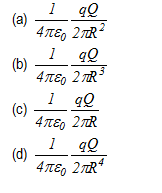Question 2
Change in radius of the ring would be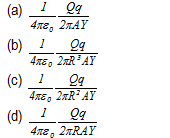where Y is the young’s modulus of the ring
Linked comprehension type (B)
A slab of uniform thickness 2d and uniform charge density ρ is lying between –d to d along x-axis and extends infinitely along y and z directions as shown below in the figure.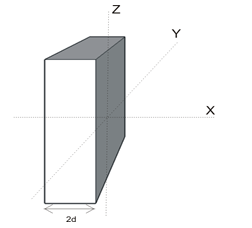Question 3
Magnitude of electric field at any point inside the slab as a function of x is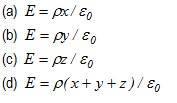Question 4
Electric field for regions outside the slab is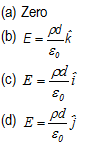Linked comprehension type (C)
Question 5
A long cylinder contains charge distributed uniformly having volume charge density ρ. (A) Electric field inside this cylinder is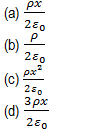Question 6
What would be the field inside the cylinder if volume charge density of the cylinder varies according to the following relation given below?
ρ=kx2
where k is a constant.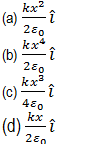Assertion reason type questions
The following questions consist of two statements, Statement I and Statement II. While answering these questions choose any of the following four responses
A. If both Statement I and Statement II are true and the reason is correct explanation of Statement I.
B. If both Statement I and Statement II are true but reason is not a correct explanation of Statement I.
C. If Statement I is true and Statement II is false.
D. If both Statement I and Statement II are false.
Question 7
Statement I: There is no electric field in the conductor
Statement II: There are plenty of free electrons in the conductors which moves in such a way, it cancel every electric field in the conductor
Question 8
Statement I: Coulombs Force is an action reaction pair
Statement II: Coulombs force is non conservative in nature

Question 9
Statement I: Two electric lines of force can never intersect
Statement II: Electric lines of force originates from negative and terminate on positive charge
Question 10
Statement I: Excess charge given to the conductor always resides on the outer surface of the conductor
Statement II: electric field is zero inside the bulk material of the conductor
Matrix Match question
Question 11
Consider the figure given below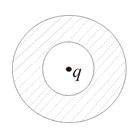A charge Q is placed inside the Spherical conductor shell
Column I
A) Charge Q is at the center of the shell
B) Charge Q is displaced from center and place at distance r from the center
C) An excess charge q is given to the shell
D) A charge q is placed near the conductor shell
Column II
X) Charge distribution is uniform at the inner surface of shell
Y) Charge distribution is non uniform at the inner surface of shell
Z) Charge distribution is uniform at the outer surface of shell
K) Charge distribution is non uniform at the outer surface of shell
Question 12
Consider the figure given below in which an infinite conductor has uniform surface charge distribution σ0 and adjacent to it is an infinite parallel layer of charge with volume charge density ρ varying according to following relation
ρ=ρ0e-αz , where z>0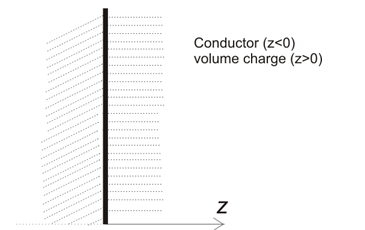Electric field due to the conductor is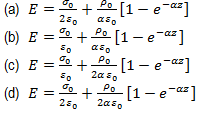Question 13
1. Two uniform infinite sheets of charge density +σ and –σ intersect at right angles. Electric field due to this configuration at any point in space is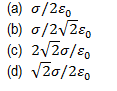Question 14
A charge Q sits at the back corner of a cube. What is the flux of electric field through the shaded surface?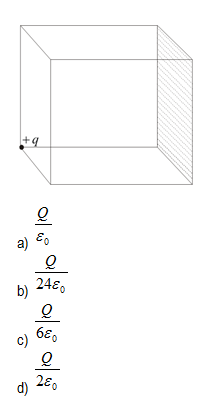Question 15
Match the column
A point charge is placed at the center of a closed spherical surface.
Φ : Electrical Flux through the closed spherical surface
E : Electric Field  through the closed spherical surface
Then Mark out the correct statement
Column I
A) If the point charge is displaced from the center but kept within the closed surface
B)  If the point charge is displaced such that it crosses the boundary of closed surface
C) If any another charge is kept outside the surface
D) If any another charge is placed inside the surface
Column II
P) Φ will change
Q) E will change
R) Φ will not change
S) E will not change
Question 16
A point charge +Q is at the origin of a coordinates system . It is surrounded by a concentric uniform distribution of charge on a spherical shell at r=R for which the total charge is -2Q
Which of the following is correct?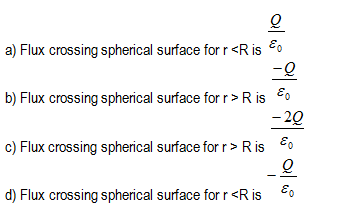Question 17
Two infinite uniform line charges of linear charge density  2 C/m lies in a XY plan  at x =+a m and  x=-a m.. A positive charge particle of charge q is placed at the origin and it is displaced by a distance k from origin towards +x axis.
Which of the following is correct
a) The charge particle will oscillate  but not harmonically for all values of  0 < k <a
b) The charge particle will oscillate  harmonically for all values of  0 < k <a
c) The charge particle will oscillate  harmonically  for k<<<a
d)  The charge particle will  not oscillate
Question 18
Two infinite uniform sheet of charge each with surface charge density σ are located at x=+a and x=-a.
Which of the following is correct?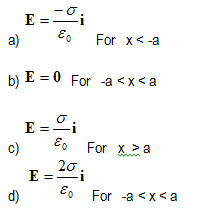Question 19
A conductor of non uniform curvature is given Q charge. Which of the following is correct
a) The charge is distributed uniformly over its volume
b) The charge is distributed uniformly over its outer surface
c) The charge has the greatest concentration on the part of greatest curvature
d) The charge has the greatest concentration on the part of least curvature
Question 20
At a  point on the axis of an electrical dipole
a) Electric field is zero
b) Electric potential is zero
c) Neither Electric field and electric potential is zero
d) Electric field is directed Perpendicular to the axis
1. (b)
2. (d)
3. (a)
4. (c)
5. (a)
6. (c)
7. (a)
8. (c)
9. (c)
10. (a)
11. A -> X ,Z : B -> Y,Z : C -> X,Z : D -> X,K
12. (b)
13. (d)
14. (b)
15. A -> Q,R : B -> P,Q : C -> Q,R : D -> P,Q
16. (a) , (b)
17. (a) , (c)
18. (a) , (b) , (c)
19. (c)
20. (c)

Solutions